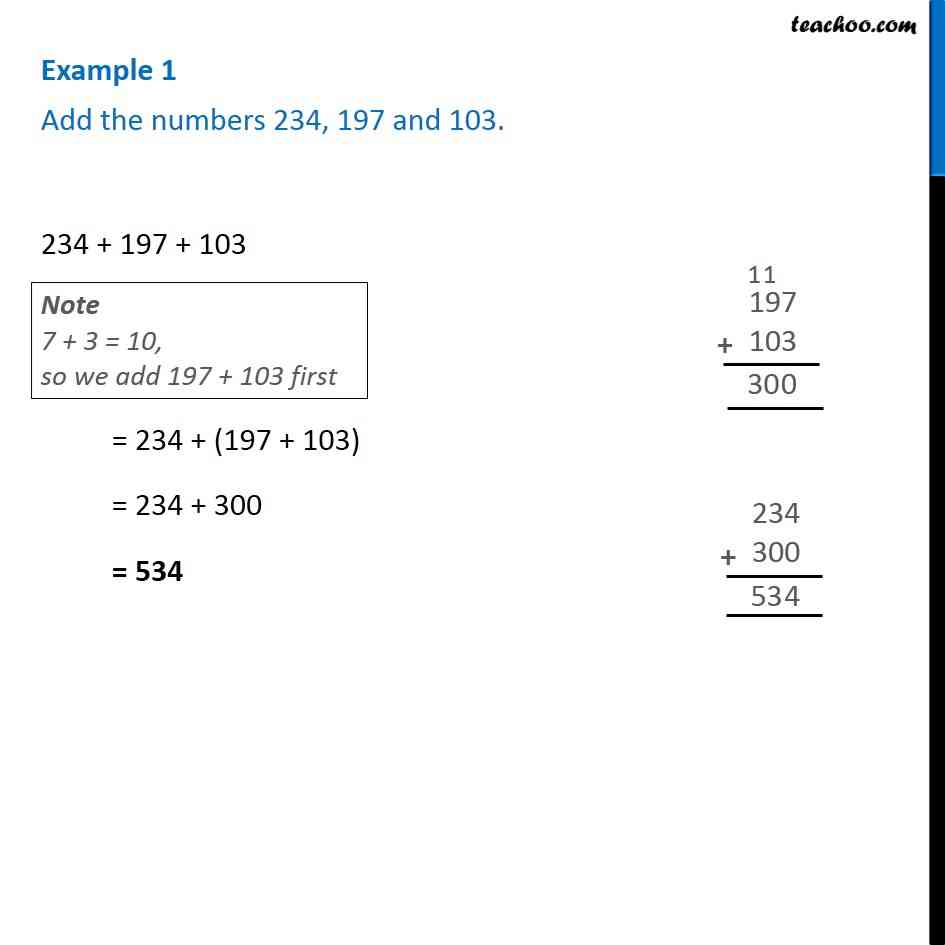1. Chapter 2 Class 6 Whole Numbers
2. Serial order wise
3. Examples

Transcript

Example 1 - Chapter 2 Class 6 Whole Numbers - NCERT Books Add the numbers 234, 197 and 103. 234 + 197 + 103 Note: 7 + 3 - 10, so we add 197 + 103 first = 234 + (197 + 103) = 234 + 300 = 534

Examples